## Example Questions

### Example Question #51 : Integers

Ifis an odd integer andis an even integer, which of the following must true of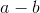?

The result will be even.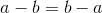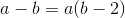The result will be odd.

We cannot draw any conclusions from the given information.

The result will be odd.

Explanation:

An even number subtracted from an odd number will always produce an odd result.

None of the other answer choices are correct.

### Example Question #2 : How To Subtract Even Numbers

Subtract: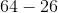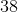Explanation:

When subtracting even numbers, we will know that the ones digit will also be an even number.

Borrow a one from the tens digit of 64, since we cannot subtract the ones digits.

After borrowing, we can then subtract.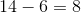Subtract the tens place.  The tens place of 64 becomes a 5.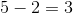Combine the two numbers.

The answer is.